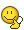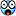# Air

Senior Members

31

## Posts posted by Air

### The Official "Introduce Yourself" Thread

Hello All! I'm 'Air'. Like Mathematics (hence most likely to be seen in the Mathematics forum) and hence have an occupation of studying it. I hope to be active member of the forum and contribute to threads. See you around.Atoms tend not to be very reactive if they:

A. have more than two orbits.

B. have complete outer orbits.

C. have a combining capacity of +2.

D. have incomplete outer orbits

??????????????????PLEASE HELP???The reactivity of an atom is determine by the vacancy (unfilled orbital) in the outermost shell of an atom. Greater vacancy implies greater reactivity (and vice versa).

### large decimals

Is it at all possible to have the number 0.0(reoccuring)1? or for that matter any other number at the end? my idea is that the number is 0.0 with an uneding string of 0s on the end, but if it did have an end, it would end in 1.

Is this possible?

If zero's are recurring, at which point would you be able to attach a zero at the end?### fringe mathematics

Hmmm... Not quite. I was just posting an alternative proof. This thread is about (Quoted OP):

I thought it would be cool to start a thread for people to post proofs/facts/interesting problems in math that warp our common sense notions about how numbers work.

### fringe mathematics

x=.999[bar]

10x=9.99[bar]

10x-x=9.99[bar]-.999[bar]

9x=9

x=1

An alternative approach (using fractions):

$\frac13 = 0.33333...$

$\frac13 \times 3 = (0.33333...) \times 3$

$1 = 0.99999...$### Why isn't zero divided by zero equal to one?

Can somebody please explain to me why zero violates common sense? Every other number including infinity, divided by itself equals one, so why doesn't the same apply to zero as well? After all, since there are numbers which are less than zero, zero itself is logically a number too, and zero does go into itself once. It just seems totally counter-intuitive that 0/0 doesn't equal one.

And while we're talking about counter-intuitive math, can someone also explain why a negative times a negative equals a positive, even though a positive times a positive doesn't equal a negative.

Please don't use fancy terms that none but a math major could understand.

Yes, normally you are told that you get the result 1 if you divide a number by itself.

If you permit this result at $\frac{0}{0}=1$ then the value of all numbers isn't unique anymore.

An example:

$5 \cdot 0 = 9 \cdot 0$. Now divide both sides of this equation by zero:

$\frac{5 \cdot 0}{0} = \frac{9 \cdot 0}{0}$. According to the assumption you can cancel out the zeros and you'll get

$5 \cdot 1= 9\cdot 1$.

Does 5 equal 9? Do you now see why zero is a special case?Well, a set of axioms must be established. I think everyone reading can agree that

Axiom 1:

0 = 1/∞

Therefore 0/0 identical to (1/∞)/(1/∞). Using (x/y)/(p/m)=(x/y)x(m/p), it must be true that 0/0 is the same as ∞/∞. This is very similar to the first question. Why isn't ∞/∞ simply one?

Well, ∞ = ∞+∞. Following on, if ∞/∞ was 1, then what is (∞+∞)/∞? Splitting the fraction, it becomes apparent that (∞+∞)/∞ = (∞/∞)+(∞/∞).

1 can never be equal to 2.

$\lim_{x \to 0^+} \ \frac 1x=+ \infty$

$\lim_{x \to 0^-} \ \frac 1x=- \infty$

$\lim_{x \to +\infty} \ \frac 1x=\lim_{x \to -\infty} \ \frac 1x=0$

$\frac{\text{Number}}{0}$ is undefined $\left(\frac{0}{0} \ne \frac{1}{\infty}\right)$. It is different from the limit.

Or... something like that. Originating from an accidentally misfiled thread, I'm trying to find the t in this equation;

$s = {v_0}t + \frac{1}{2}at^2$

Normally I'd try to shuffle the values around, adding, subtracting, dividing etc. until I ended up with what I wanted, but I've long since forgotten how to deal with multiple exponents and barely remembering the terminology {let alone in English} didn't help finding an answer either.

Would someone be so kind as to give me a pointer or two how to deal with this?

When you rearrange the formula, you are able to get it into the form $ax^2 + bx + c =0$. $s = {v_0}t + \frac{1}{2}at^2 \rightarrow \frac12 at^2 + {v_0}t - s = 0$. This implies:

$a = \left(\frac12 a\right), \ b={v_0}, \ c={-s}$.

For these type of formula, known as the quadratic equation (Highest power is 2), you can use the quadratic equation to find the value of $x$. The quadratic formula is:

$x= \frac{-b \pm \sqrt{b^2 - 4ac}}{2a}$

### Assignment help.

This is similar to my question about Gay-Lussac.

I just need to know what Albert Einsteins specialization is physics was.

He is best known for his theory of relativity and specifically mass–energy equivalence, $E = mc^2$. Einstein received the 1921 Nobel Prize in Physics "for his services to Theoretical Physics, and especially for his discovery of the law of the photoelectric effect".

### Most Elegant Math Equations Ever

In your opinion, what equations, solutions, or sets are the most elegant math equations ever solved or conceived of?

Personally, I like Euler's identity. That is, e^(i*pi) +1 = 0. The way it is proven is also something to marvel at too, since it comes directly out of complex analysis.

I like Euler's Identity too, $e^{\pi i} + 1 = 0$. It's one of those equations which would strike people's mind when we say 'Mathematics' just as when we say 'Physics', people's mind will get Einstein's equation $e=mc^2$.

×